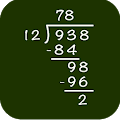# Math: Long Division APK

## com.epsilon.division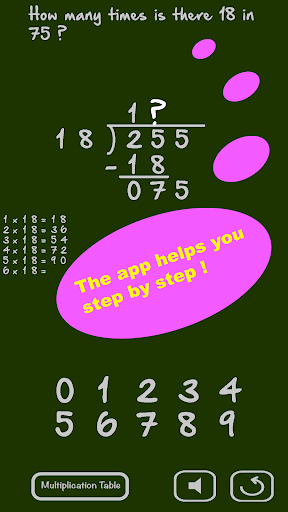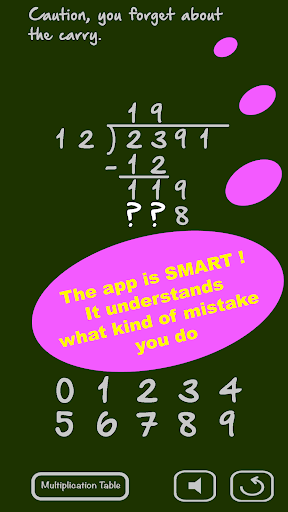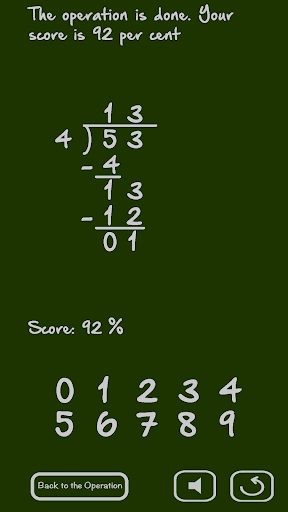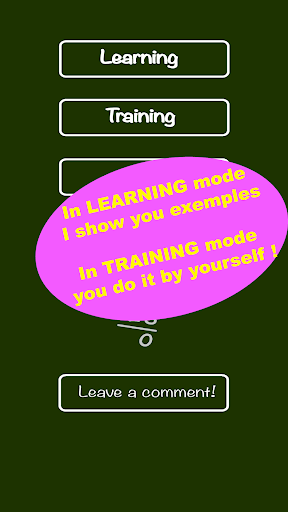The application will teach you how to do a long division. The application is smart: it tries to understand your mistakes and give you appropriate indications.

The long division is a fundamental concept of math. It includes the division of course, but also the multiplication, the subtraction and the multiplication tables, all math operations. Mastering the long division means mastering all these technics of math.

The application proposes two modes: training and learning.

In learning mode, the application shows you how to do the long division step by step. Each step of the math exercise is explained and detailed. You can choose the numbers, divisor and dividend, or let the application randomly choose the division to do.

In training mode, you do the long division step by step by yourself. The application detects the mistakes, such as forgetting the carry. It gives you indications if you need all along math exercises.

Several levels are possible for math exercises. The easy level proposes simple numbers, for the early learning of division. The following levels proposes more and more difficult exercises. One can choose between integer long division or decimal numbers, with or without intermediate subtraction.

The application computes a score from time and mistakes during the division. This gives you motivation to train and let you see the progression in the long division.

Note: The application uses vocal synthesis (it speaks) to comment the division during math exercises. On some devices, it will propose to you to install upgrades of vocal synthesis if you want to get better diction quality.

#### What’s New

long division for all